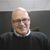Announcements
MAINTENANCE ALERT: Dec. 12th starting 9 AM CET. Community will be read-only. GET DETAILS
cancel
Showing results for
Did you mean:Contributor III

## Use selection as parameter in expression

Hi,

I have selected a date from a multibox and would like to use set analysis with the whole set (1) in order to sum a field that is

SUM({1<datefield={Highest value less than date selection}>}Sumfield)

Is that possible?

1 Solution

Accepted SolutionsMVP

Missed a parenthesis

Sum({1<datefield = {"\$(=Date(Max({<datefield = {[\$(='<' & Date(Min(datefield)))]}>} datefield)))"}>} Sumfield)

7 RepliesChampion III

May be this?

= SUM({<datefield= { [\$(=GetFieldSelections(YourfieldselectionFieldname))] } >}Sumfield)Contributor III
Author

Almost thereIt also needs to be latest date less than the selection.MVP

May be using nested set analysis

Sum({1<datefield = {"\$(=Date(Max({<datefield = {[\$(='<' & Date(Min(datefield))]}>}datefield)))"}>} Sumfield)MVP

Missed a parenthesis

Sum({1<datefield = {"\$(=Date(Max({<datefield = {[\$(='<' & Date(Min(datefield)))]}>} datefield)))"}>} Sumfield)Champion III

My bad completely misunderstood the question. Try sunny's expression and let us know. 🙂Partner - Champion III

A search string within a set expression will do:

Sum( {<Date={'\$(=Max({<Date={"<\$(=Date)"}>} Date))'}>} Sales)

Replace Date with datefield and Sales with Sumfield above ...Contributor III
Author

Thank you!Community Browser